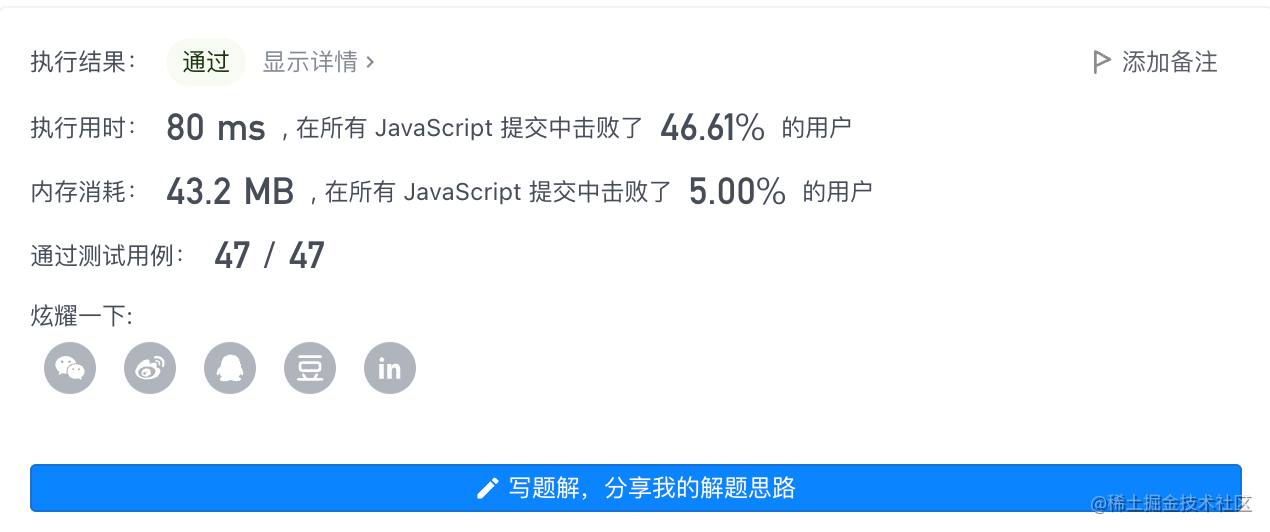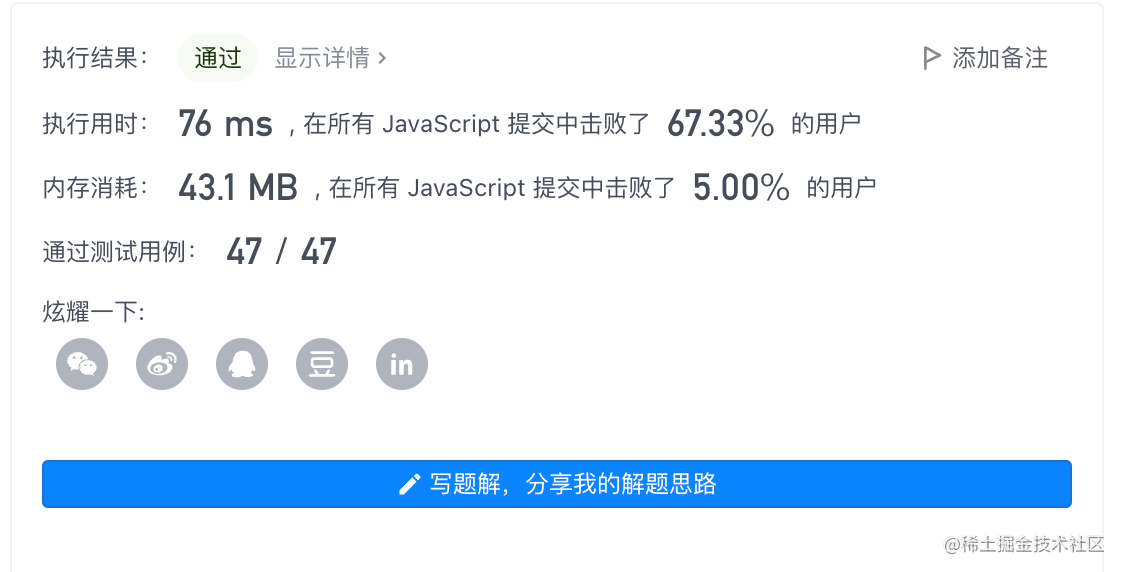2022-11-1700

## 目录

1. 题目
2. 解一：哈希表
3. 投票算法Boyer-Moore

# 1. 题目

``````输入： [3,2,3]

``````

``````输入： [2,2,1,1,1,2,2]

``````

# 2. 解一：哈希表

```javascript```/**
* @param {number[]} nums
* @return {number}
*/
var majorityElement = function(nums) {
const a = []
const n = nums.length >> 1
for(let index in nums) {
a[nums[index]] = a[nums[index]] !== undefined ? ++a[nums[index]] : 1
if(a[nums[index]] > n) {
return nums[index]
}
}
};

``````

• 时间复杂度: O(n)
• 空间复杂度: O(n)# 3. 投票算法Boyer-Moore

• 我们维护一个候选众数 `candidate` 和它出现的次数 `count`。初始时 `candidate` 可以为任意值，`count` 为 `0`
• 我们遍历数组 `nums` 中的所有元素，对于每个元素 `nums`，在判断 `x` 之前，如果 `count` 的值为 `0`，我们先将 `x` 的值赋予 `candidate`，随后我们判断 `x`
• 如果 `x``candidate` 相等，那么计数器 `count` 的值增加 `1`
• 如果 `x``candidate` 不等，那么计数器 `count` 的值减少 `1`
• 因为题目假设数组总是存在多数元素，所以在遍历完成后，`candidate` 即为整个数组的众数。
```javascript```/**
* @param {number[]} nums
* @return {number}
*/
var majorityElement = function(nums) {
let count = 0, candidate
for(let i in nums) {
if(count === 0) {
candidate = nums[i]
}
count =  (nums[i] === candidate) ? ++count : --count
}
return candidate
};
``````

• 时间复杂度： O(n)
• 空间复杂度： O(1)